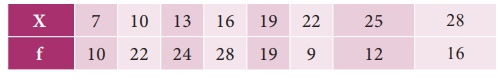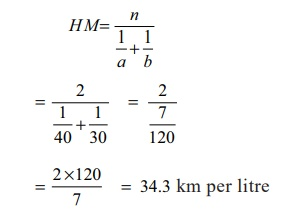Home | | Business Maths 11th std | Relationship among the averages

# Relationship among the averages

In any distribution when the original items differ in size, the values of AM, GM and HM would also differ and will be in the following order AM â‰¥ GM â‰¥ HM

Relationship among the averages

In any distribution when the original items differ in size, the values of AM, GM and HM would also differ and will be in the following order

AM â‰¥ GM â‰¥ HMExample 8.13

Verify the relationship among AM, GM and HM for the following dataSolution:Example 8.14

Aâ€™s scooter gives an average of 40km a litre while Bâ€™s scooter gives an average of 30km a litre . Find out the mean, if

(i) each one of them travels 120 km.

(ii) the petrol consumed by both of them is 2 litres per head.

Solution:

(i)  Here the distance is constant. Hence harmonic mean is appropriate.(ii) Here the quantity of petrol consumed is fixed i.e 2 litres. Here the arithmetic mean will give the correct answer.âˆ´ Average speed = 35 km per litre.

Example 8.15

A person purchases tomatoes from each of the 4 places at the rate of 1kg., 2kg., 3kg., and 4kg. per rupee respectively .On the average, how many kilograms has he purchased per rupee?

Solution:

Since we are given rate per rupee, harmonic mean will give the correct answer.Tags : Measures of central tendency , 11th Business Mathematics and Statistics(EMS) : Chapter 8 : Descriptive statistics and probability
Study Material, Lecturing Notes, Assignment, Reference, Wiki description explanation, brief detail
11th Business Mathematics and Statistics(EMS) : Chapter 8 : Descriptive statistics and probability : Relationship among the averages | Measures of central tendency